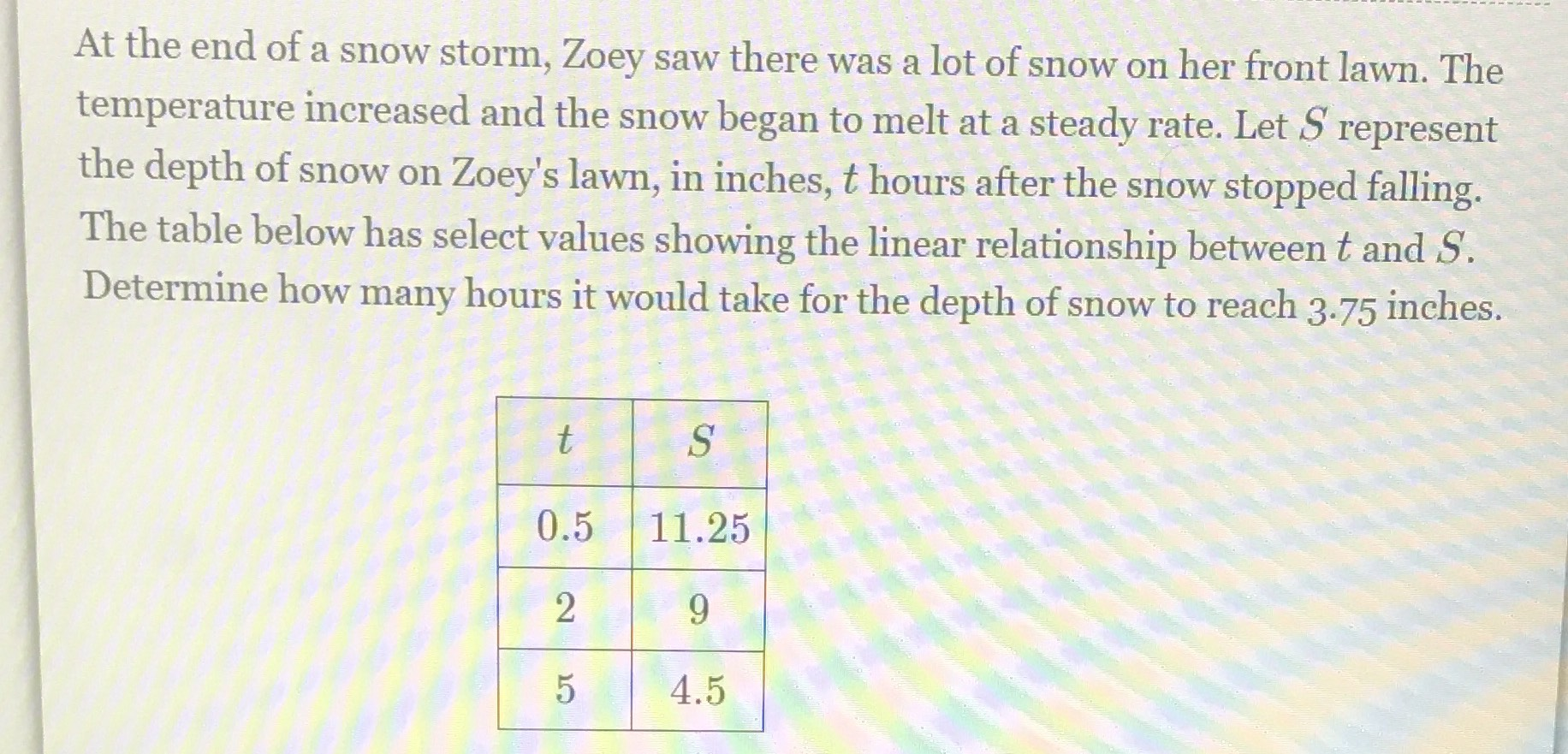### ¿Todavía tienes preguntas de matemáticas?

Pregunte a nuestros tutores expertos
Algebra
PreguntaAt the end of a snow storm, Zoey saw there was a lot of snow on her front lawn. The temperature increased and the snow began to melt at a steady rate. Let $$S$$ represent the depth of snow on Zoey's lawn, in inches, $$t$$ hours after the snow stopped falling. The table below has select values showing the linear relationship between $$t$$ and $$S$$ . Determine how many hours it would take for the depth of snow to reach $$3.75$$ inches.